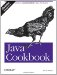# Recipe 5.9 Converting Between Binary, Octal, Decimal, and Hexadecimal

#### Problem

You want to display an integer as a series of bits for example, when interacting with certain hardware devices. You want to convert a binary number or a hexadecimal value into an integer.

#### Solution

The class java.lang.Integer provides the solutions. Use toBinaryString( ) to convert an integer to binary. Use valueOf( ) to convert a binary string to an integer:

`// BinaryDigits.java  String bin = "101010";  System.out.println(bin + " as an integer is " + Integer.valueOf(bin, 2));  int i = 42;  System.out.println(i + " as binary digits (bits) is " +       Integer.toBinaryString(i));`

This program prints the binary as an integer, and an integer as binary:

`\$ java BinaryDigits 101010 as an integer is 42 42 as binary digits (bits) is 101010 \$`

#### Discussion

Integer.valueOf( ) is more general than binary formatting. It also converts a string number from any radix to int, just by changing the second argument. Octal is base 8, decimal is 10, hexadecimal 16. Going the other way, the Integer class includes toBinaryString( ) , toOctalString( ), and toHexString( ).

The String class itself includes a series of static methods, valueOf(int), valueOf(double), and so on, that also provide default formatting. That is, they return the given numeric value formatted as a string.Java Cookbook, Second Edition
ISBN: 0596007019
EAN: 2147483647
Year: 2003
Pages: 409
Authors: Ian F Darwin

Similar book on Amazon